View markdown source on GitHub

# Convolutional neural networks (CNN) Deep Learning - Part 3

## Questions

• What is a convolutional neural network (CNN)?

• What are some applications of CNN?

## Objectives

• Understand the inspiration behind CNN and learn the CNN architecture

• Learn the convolution operation and its parameters

• Learn how to create a CNN using Galaxy’s deep learning tools

• Solve an image classification problem on MNIST digit classification dataset using CNN in Galaxy

## Requirements

last_modification Last modification: Oct 1, 2023

# What is a convolutional neural network (CNN)?

Speaker Notes

What is a convolutional neural network (CNN)?

# Convolutional Neural Network (CNN)

• Increasing popularity of social media in past decade
• Image and video processing tasks have become very important
• FNN could not scale up to image and video processing tasks
• CNN specifically tailored for image and video processing tasks

# Feedforward neural networks (FNN)

• In FNN all nodes in a layer connected to all nodes in next layer
• Each connection has a weight, must be learned by learning algorithm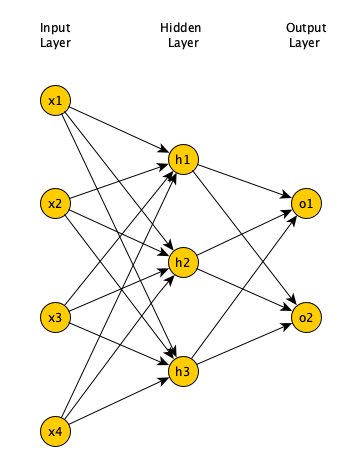# Limitations of FNN

• If input is 64 pixel by 64 pixel grayscale image
• Each grayscale pixel represented by 1 value, usually between 0 to 255
• Where 0 is black, 255 is white, and values in between are shades of gray
• Since each grayscale pixel represented by 1 value, we say channel size is 1
• Image represented by 64 x 64 x 1 = 4,096 values (rows x columns x channels)
• Hence, input layer of FNN has 4096 nodes
• Lets assume next layer has 500 nodes
• Since FNN fully connected, we have 4,096 x 500 = 2,048,000 weights

# Limitations of FNN

• For complex problems, we need multiple hidden layers in our FNN
• Compunds the problem of having many weights
• Having too many weights
• Makes learning more difficult as dimension of search space is increased
• Makes training more time/resource consuming
• Increases the likelihood of overfitting
• Problem is further compunded for color images
• Each pixel in color image represented by 3 values (RGB color mode)
• Since each pixel represented by 3 values, we say channel size is 3
• Image represented by 64 x 64 x 3 = 12,288 values (rows x columns x channels)
• Number of weights is now 12,288 x 500 = 6,144,000

# Limitations of FNN

• Clear that FNN cannot scale to larger images (Too many weights)
• Another problem with FNN
• 2D image represented as 1D vector in input layer
• Any spatial relationship in the data is ignored

# Inspiration for CNN

• In 1959 Hubel & Wiesel did an experiment to understand how visual cortex of brain processes visual info
• Recorded activity of neurons in visual cortex of a cat
• While moving a bright line in front of the cat
• Some cells fired when bright line is shown at a particular angle/location
• Called these simple cells
• Other cells fired when bright line was shown regardless of angle/location
• Seemed to detect movement
• Called these complex cells
• Seemed complex cells receive inputs from multiple simple cells
• Have an hierarchical structure
• Hubel and Wiesel won Noble prize in 1981

# Inspiration for CNN

• Inspired by complex/simple cells, Fukushima proposed Neocognitron (1980)
• Hierarchical neural network used for handwritten Japanese character recognition
• First CNN, had its own training algorithm
• In 1989, LeCun proposed CNN that was trained by backpropagation
• CNN got popular when outperformed other models at ImageNet Challenge
• Competition in object classification/detection
• On hundreds of object categories and millions of images
• Run annually from 2010 to present
• Notable CNN architectures that won ImageNet challenge
• AlexNet (2012), ZFNet (2013), GoogLeNet & VGG (2014), ResNet (2015)

# Architecture of CNN

• A typical CNN has 4 layers
• Input layer
• Convolution layer
• Pooling layer
• Fully connected layer
• We will explain a 2D CNN here
• Same concepts apply to a 1 (or 3) dimensional CNN

# Input layer

• Example input a 28 pixel by 28 pixel grayscale image
• Unlike FNN, we do not “flatten” the input to a 1D vector
• Input is presented to network in 2D as 28 x 28 matrix
• This makes capturing spatial relationships easier

# Convolution layer

• Composed of multiple filters (kernels)
• Filters for 2D image are also 2D
• Suppose we have a 3 by 3 filter (9 values in total)
• Values are randomly set to 0 or 1
• Convolution: placing 3 by 3 filter on the top left corner of image
• Multiply filter values by pixel values, add the results
• Move filter to right one pixel at a time, and repeat this process
• When at top right corner, move filter down one pixel and repeat process
• Process ends when we get to bottom right corner of image

# 3 by 3 Filter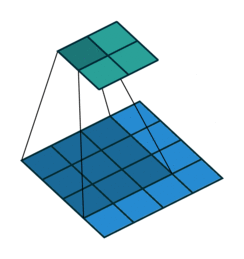# Convolution operator parameters

• Filter size
• Stride
• Dilation
• Activation function

# Filter size

• Filter size can be 5 by 5, 3 by 3, and so on
• Larger filter sizes should be avoided
• As learning algorithm needs to learn filter values (weights)
• Odd sized filters are preferred to even sized filters
• Nice geometric property of all input pixels being around output pixel

• After applying 3 by 3 filter to 4 by 4 image, we get a 2 by 2 image – Size of the image has gone down
• If we want to keep image size the same, we can use padding
• We pad input in every direction with 0’s before applying filter
• If padding is 1 by 1, then we add 1 zero in evey direction
• If padding is 2 by 2, then we add 2 zeros in every direction, and so on

# 3 by 3 filter with padding of 1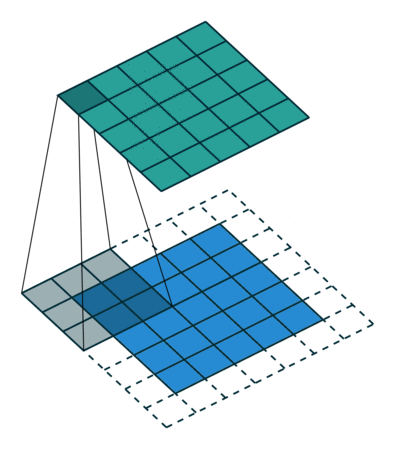# Stride

• How many pixels we move filter to the right/down is stride
• Stride 1: move filter one pixel to the right/down
• Stride 2: move filter two pixels to the right/down

# 3 by 3 filter with stride of 2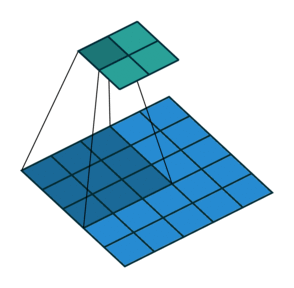# Dilation

• When we apply 3 by 3 filter, output affected by pixels in 3 by 3 subset of image
• Dilation: To have a larger receptive field (portion of image affecting filter’s output)
• If dilation set to 2, instead of contiguous 3 by 3 subset of image, every other pixel of a 5 by 5 subset of image affects output

# 3 by 3 filter with dilation of 2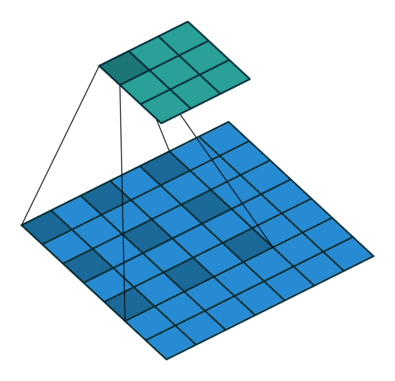# Activation function

• After filter applied to whole image, apply activation function to output to introduce non-linearlity
• Preferred activation function in CNN is ReLU
• ReLU leaves outputs with positive values as is, replaces negative values with 0

# Relu activation function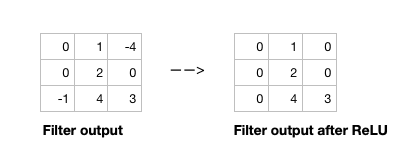# Single channel 2D convolution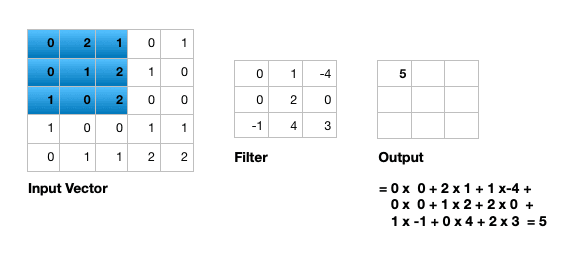# Triple channel 2D convolution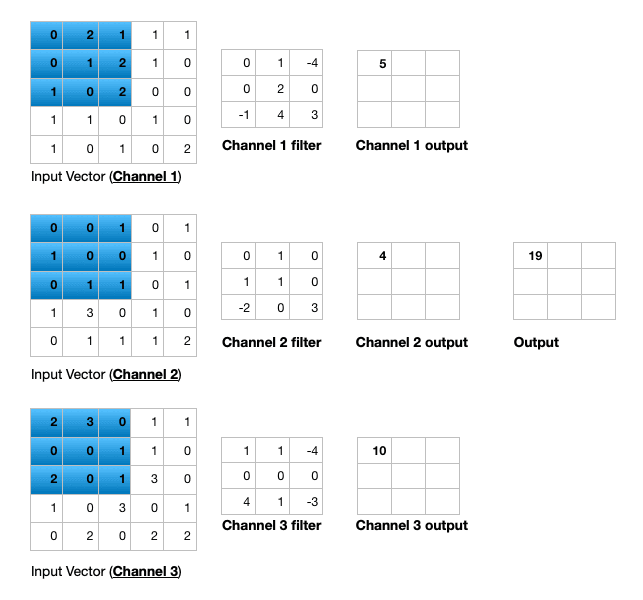# Triple channel 2D convolution in 3D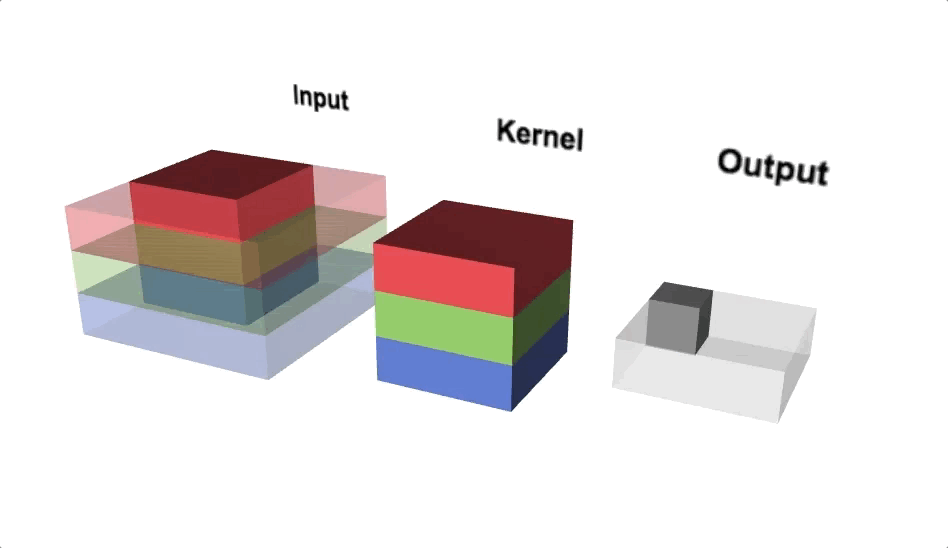# Change channel size

• Output of a multi-channel 2D filter is a single channel 2D image
• Applying multiple filters results in a multi-channel 2D image
• E.g., if input image is 28 x 28 x 3 (rows x columns x channels)
• We apply a 3 x 3 filter with 1 x 1 padding, we get a 28 x 28 x 1 image
• If we apply 15 such filters, we get a 28 x 28 x 15
• Number of filters allows us to increase or decrease channel size

# Pooling layer

• Pooling layer performs down sampling to reduce spatial dimensionality of input
• This decreases number of parameters
• Reduces learning time/computation
• Reduces likelihood of overfitting
• Most popular type is max pooling
• Usually a 2 x 2 filter with a stride of 2
• Returns maximum value as it slides over input data

# Fully connected layer

• Last layer in a CNN
• Connect all nodes from previous layer to this fully connected layer
• Which is responsible for classification of the image

# An example CNN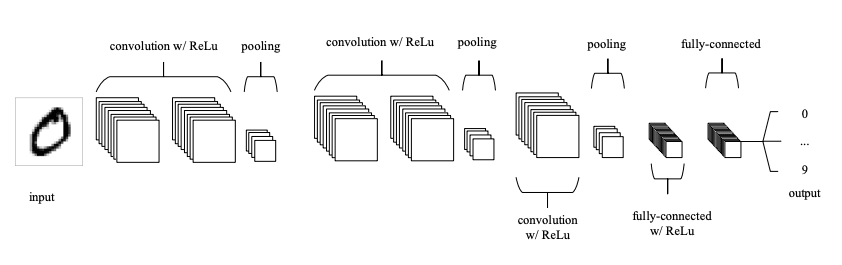# An example CNN

• A typical CNN has several convolution plus pooling layers
• Each responsible for feature extraction at different levels of abstraction
• E.g., filters in first layer detect horizontal, vertical, and diagonal edges
• Filters in the next layer detect shapes
• Filters in the last layer detect collection of shapes
• Filter values randomly initialized, learned by learning algorithm
• CNN not only do classification, but can also automatically do feature extraction
• Distinguishes CNN from other classification techniques (like Support Vector Machines)

# MNIST dataset

• MNIST dataset of handwritten digits
• Composed of training set of 60,000 and test set of 10,000 images
• Digits have been size-normalized/centered in a fixed-size image (28 by 28 pixels)
• Images are grayscale
• Each pixel is represented by a number between 0 and 255
• 0 for black, 255 for white, and other values for shades of gray
• MNIST dataset is a standard image classification dataset

# Classification of MNIST images with CNN

• We define a CNN and train it using MNIST dataset training data
• Goal is to learn a model such that given image of a digit we predict the digit (0 to 9)
• We then evaluate the trained CNN on test dataset and plot the confusion matrix

# For references, please see tutorial’s References section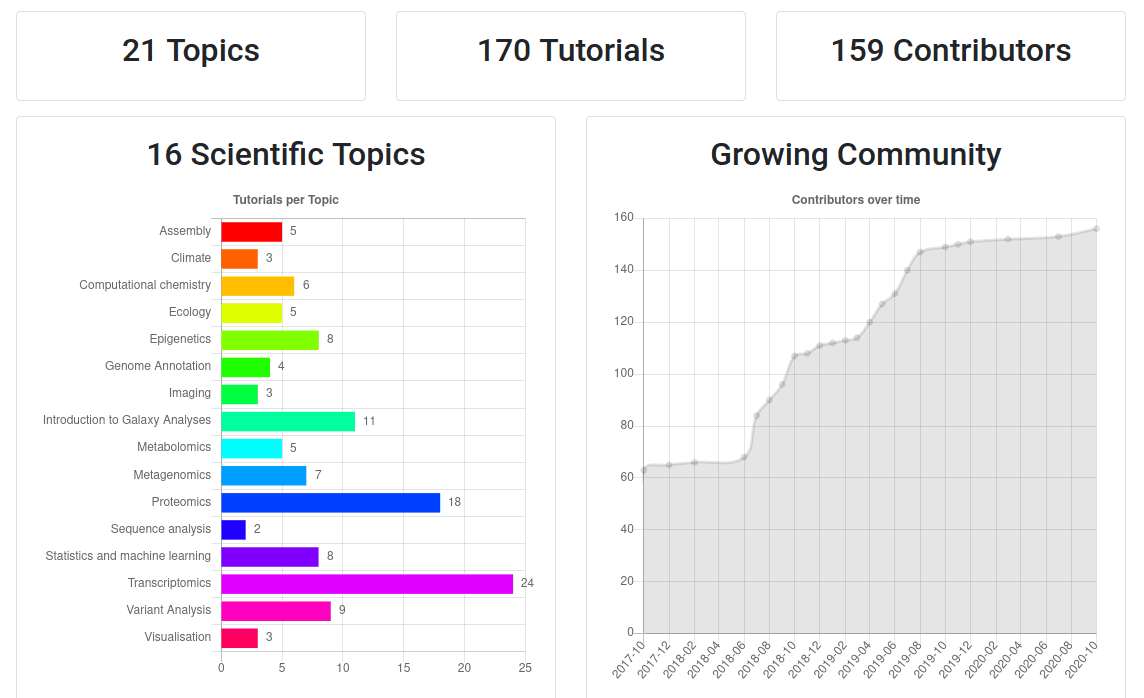Speaker Notes

• If you would like to learn more about Galaxy, there are a large number of tutorials available.
• These tutorials cover a wide range of scientific domains.

# Getting Help

Speaker Notes

• If you get stuck, there are ways to get help.
• Or you can chat with the community on Gitter.

# Join an event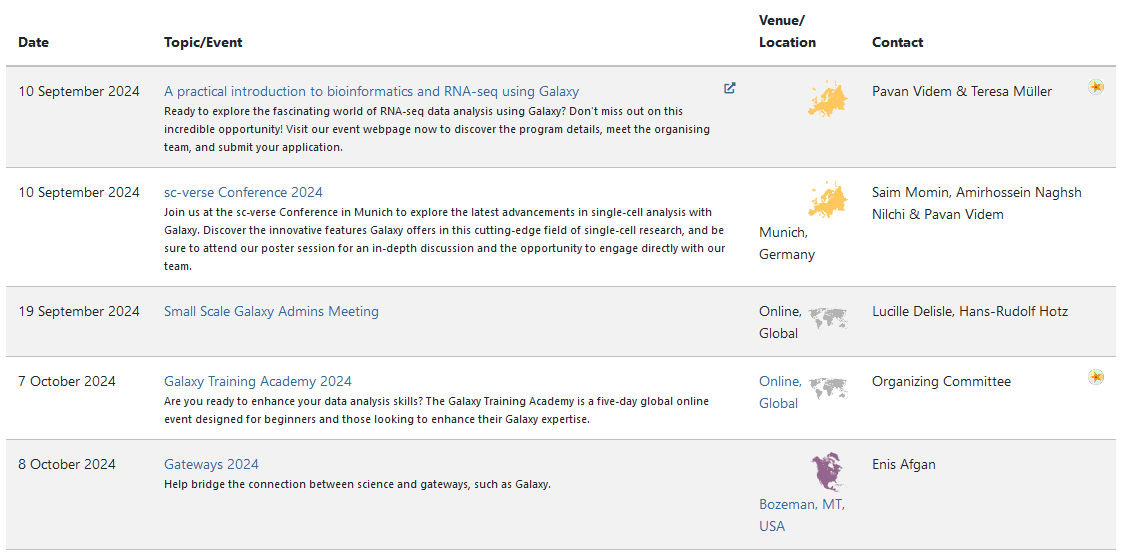Speaker Notes

• There are frequent Galaxy events all around the world.
• You can find upcoming events on the Galaxy Event Horizon.

## Thank you!

This material is the result of a collaborative work. Thanks to the Galaxy Training Network and all the contributors!This material is licensed under the Creative Commons Attribution 4.0 International License.